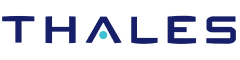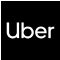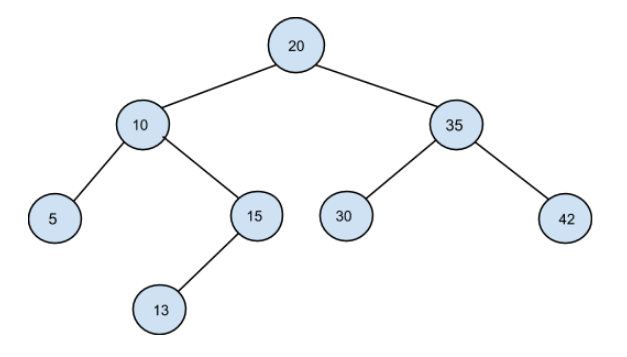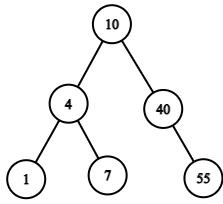New update is available. Click here to update.
Topics

# Preorder traversal of a BST

Moderate0/80
Average time to solve is 15m+10 more companies

## Problem statement

You have been given an array/list 'PREORDER' representing the preorder traversal of a BST with 'N' nodes. All the elements in the given array have distinct values.

Your task is to construct a binary search tree that matches the given preorder traversal.

A binary search tree (BST) is a binary tree data structure that has the following properties:

``````• The left subtree of a node contains only nodes with data less than the node’s data.
• The right subtree of a node contains only nodes with data greater than the node’s data.
• Both the left and right subtrees must also be binary search trees.
``````

Note:

``````It is guaranteed that a BST can be always constructed from the given preorder traversal. Hence, the answer will always exist.
``````
Example:
``````From PREORDER = [20, 10, 5, 15, 13, 35, 30, 42] , the following BST can be constructed:
``````Detailed explanation ( Input/output format, Notes, Images )
Constraints :
``````1 <= T <= 100
1 <= N <= 5000
0 <= data <= 10^5

Where 'data' denotes data contained in the nodes of the binary search tree.

Time Limit: 1 sec
``````
Sample Input 1:
``````1
6
10 4 3 7 40 55
``````
Sample Output 1:
``````3 5 7 10 40 50
``````
Explanation For Sample Output1:
``````From the given preorder traversal, the following BST can be constructed:
````````````The inorder traversal of the given BST is [1, 4, 7, 10, 40, 55].
``````
Sample Input 2:
``````2
7
15 10 7 13 21 20 25
3
1 2 4
``````
Sample Output 2:
``````7 10 13 15 20 21 25
1 2 4
``````Console